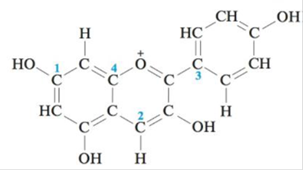Chapter 4, Problem 106CWPChemistry: An Atoms First Approach

2nd Edition
Steven S. Zumdahl + 1 other
ISBN: 9781305079243

Solutions

Chapter
SectionChemistry: An Atoms First Approach

2nd Edition
Steven S. Zumdahl + 1 other
ISBN: 9781305079243
Textbook Problem

Pelargondin is the molecule responsible for the red color of the geranium flower. It also contributes to the color of ripe strawberries and raspberries. The structure of pelargondin is:How many σ and π bonds exist in pelargondin? What is the hybridization of the carbon atoms marked 1–4?

Interpretation Introduction

Interpretation: The number of pi (π) and sigma (σ) bonds present in the given structure are to be calculated. The hybridization of the marked carbon atoms is to be stated.

Concept introduction: Sigma bonds are the single bonds present between atoms in a compound. The second bonding that occurs between the atoms is known as the pi bonding.

The hybridization of an atom can be obtained by finding its steric number. The sum of the numbers of atoms bonded to the required atom and the number of lone pairs the atom has is known as the steric number.

If the steric number is 4 , the atom is sp3 hybridized.

If the steric number is 3 , the atom is sp2 hybridized.

If the steric number is 2 , the atom is sp hybridized.

To determine: The number of pi (π) and sigma (σ) bonds and the hybridization of the carbon atoms marked in the structure.

Explanation

Sigma bonds are the single bonds present between atoms in a compound. The second bonding that occurs between the atoms is known as the pi bonding.\

Figure 1

The number of sigma bonds present are 32

Still sussing out bartleby?

Check out a sample textbook solution.

See a sample solution

The Solution to Your Study Problems

Bartleby provides explanations to thousands of textbook problems written by our experts, many with advanced degrees!

Get Started